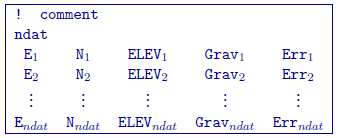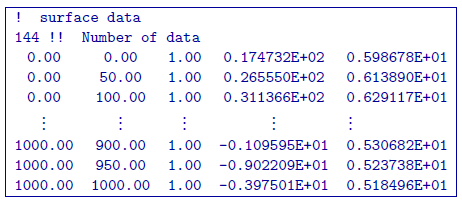# 5.1.4. Gravity observations: GIF file¶

This file is used to specify the observation locations and observed gravity anomalies with estimated standard deviation. The output of the forward modelling program gzfor3d has the same structure except that the column of standard deviations for the error is omitted. Lines starting with ! are comments. The following is the GIF-formatted file structure of a gravity observations file:Parameter definitions:

• ndat: Number of observations.

• E, N, ELEV: Easting, northing and elevation of the observation, measured in meters. Elevation should be above the topography for surface data, and below the topography for borehole data. The observation locations can be listed in any order.

• Grav $$_i$$: Anomalous gravity of ith datum measured in mGal.

• Err $$_i$$: Standard deviation of Grav$$_n$$. This represents the absolute error. It must be positive and non-zero.

NOTE: It should be noted that the data are extracted anomalies which are derived by removing the regional from the field measurements. Furthermore, the inversion program assumes that the anomalies are produced by a density contrast distribution in g/cm $$^3$$ with mesh cells in meters. Therefore, it is crucial that the data be prepared in mGal.

## 5.1.4.1. Example¶